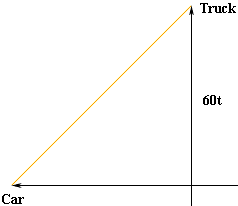Quandaries and Queries Name: Weisu secondary student I have questions about three word problems and one regular problem, all dealing with derivatives. Find all points on xy=exy where the tangent line is horizontal. The width x of a rectangle is decreasing at 3 cm/s, and its length y is increasing at 5 cm/s. At what rate is its area A changing when x=10 and y=15? A car and a truck leave the same intersection, the truck heading north at 60 mph and the car heading west at 55 mph. At what rate is the distance between the car and the truck changing when the car and the truck are 30 miles and 40 miles from the intersection, respectively? The production P of a company satisfies the equation P=x2 + 0.1xy + y2, where x and y are the inputs. At a certain period x=10 units and y=8 units. Estimate the change in y that should be made to set up a decrease of 0.5 in the input x so that the production remains the same. If you could just give me some hints on these questions, I'd really appreciate it. Thanks! -Weisu Hi Weisu, I can give you a hand on a couple of these. You can consider y as a function of x and differentiate both sides with respect to x, and then set y' equal to zero to find where the tangent is horizontal. When you do this you get x = 0 or y = 0, neither of which make sense in the original expression xy=exy. In fact, on closer examination nothing seems to make sense in this problem. There are no numbers x and y for which xy=exy. There is no graph! I started with a diagram.Since the truck is travelling at 60 mph, if your clock starts when the truck leaves the intersection then at time t hours the truck has travelled 60t miles. In half an hour the truck will have travelled 30 miles but the car will only travel 27.5 miles in half an hour. If the car is 40 miles from the intersection when the truck is 30 miles from the intersection then the car must have been 40-27.5=12.5 miles from the intersection when you started your clock. Thus, at time t hours the car is 55t + 12.5 miles from the intersection. Now you can use Pythagoras' Theorem to find the square of the distance D between the car and the truck. D2 = (60t)2 + (55t + 12.5)2 Can you complete it now? Penny Go to Math Central